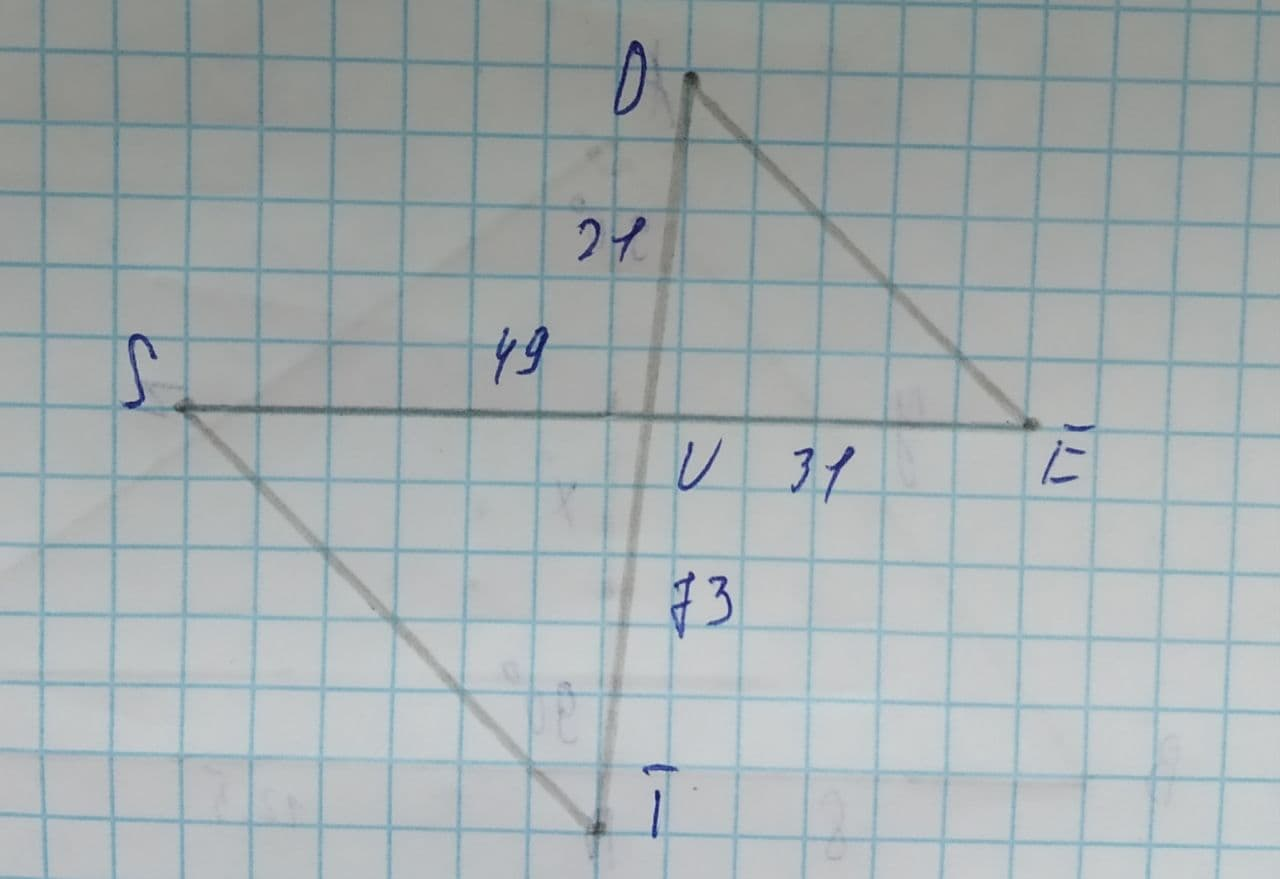# Are the two triangles similar? If so, by what similarity shortcut?slaggingV 2021-08-10 Answered
Are the two triangles similar? If so, by what similarity shortcut?SSS
SAS
AA
Not Similar
You can still ask an expert for help

• Questions are typically answered in as fast as 30 minutes

Solve your problem for the price of one coffee

• Math expert for every subject
• Pay only if we can solve itfaldduE
Step 1
For any two triangles to be similar
(1) The corresponding angles of both the triangles should be equal
(2) The corresponding sides of both the triangles should be in proportion to each other.
Step 2
In the given triangles, $\mathrm{\angle }SUT=\mathrm{\angle }DUE$ (vertically opposite angles)
The ratio of sides,
$\frac{SU}{UD}=\frac{49}{21}2.333$
$\frac{TU}{UE}=\frac{73}{31}2.354$
As we see that the sides are not in proportion, so the triangles are not similar.